Courses

# Test: General Properties Of 15Th Group (Nitrogen & Phosphorus)

## 21 Questions MCQ Test Chemistry Class 12 | Test: General Properties Of 15Th Group (Nitrogen & Phosphorus)

Description
This mock test of Test: General Properties Of 15Th Group (Nitrogen & Phosphorus) for Class 12 helps you for every Class 12 entrance exam. This contains 21 Multiple Choice Questions for Class 12 Test: General Properties Of 15Th Group (Nitrogen & Phosphorus) (mcq) to study with solutions a complete question bank. The solved questions answers in this Test: General Properties Of 15Th Group (Nitrogen & Phosphorus) quiz give you a good mix of easy questions and tough questions. Class 12 students definitely take this Test: General Properties Of 15Th Group (Nitrogen & Phosphorus) exercise for a better result in the exam. You can find other Test: General Properties Of 15Th Group (Nitrogen & Phosphorus) extra questions, long questions & short questions for Class 12 on EduRev as well by searching above.
QUESTION: 1

### Hot conc. H2SO4 acts as moderately strong oxidising agent. It oxidises both metals and nonmetals. Which of the following element is oxidised by conc. H2SO4 into two gaseous products?

Solution:

C element is oxidised by conc. H2SO4 into two gaseous products.

QUESTION: 2

### Correct order of 2nd ionisation energy of C, N, O and F is

Solution:

The second ionization energy refers to the energy required to remove the electron from the corresponding mono-valent cation of the respective atom. The atoms: C, N, O and F belong to 2nd period of the periodic table.

Just like second ionization energy like the first IE is affected by size, effective nuclear charge, type of orbital from which the electron is being removed and electronic configuration.

It is expected to increase from left to right in the periodic table with decrease in the atomic size. However oxygen has greater second ionization energy than fluorine and also nitrogen.

Reason: Since Oxygen atom gets stable electronic configuration, 2s22p3 after removing one electron, the O+ shows greater ionization energy than F+ as well as N+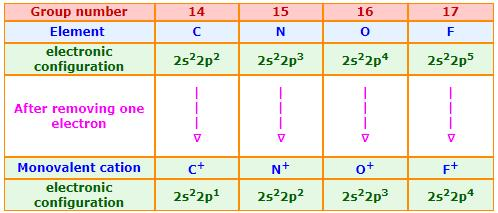QUESTION: 3

### Which trend enthalpy is correct ?

Solution: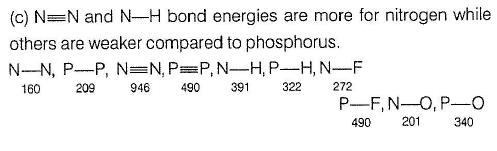QUESTION: 4

Boiling Point of liquid nitrogen is

Solution:

Liquid nitrogen is a cryogenic fluid that can cause rapid freezing on contact with living tissue. When appropriately insulated from ambient heat, liquid nitrogen can be stored and transported, for example in vacuum flasks. The temperature is held constant at 77 K by slow boiling of the liquid, resulting in the evolution of nitrogen gas.

QUESTION: 5

Oxidation of ammonia with Cuo produce a gaseous chemical which can also be obtained by

Solution:

Actually when ammonia is passed through a solution of calcium hypochlorite (bleaching powder), bromide water or passed over heated Cu oxide, it is oxidized to dinitrogen gas. 2NH3+3CuO = 3Cu+3H2O+N2,
With excess NH3, 8NH3+3Cl2 = 6NH4Cl+N2

QUESTION: 6

Which of the following molecular species has unpaired electron(s) ?

Solution:contains two unpaired electrons and is paramagnetic in nature. On the other hand,andcontains all paired electrons and are diamagnetic in nature.

QUESTION: 7

Which is the correct order w.r.t the given property?

Solution:

Only electro negativity of the given 4 decreases down the group whilst all the others increase or stay the same down the group.
Hence B is correct.

QUESTION: 8

The ratio of bond pairs and lone pairs in a P4 molecule is

Solution:

(i) It has six P-P single bonds.
(ii)it has four lone pair of electrons. Ratio of 6/4=3/2 or 3:2

*Multiple options can be correct
QUESTION: 9

One or More than One Options Correct Type
This section contains 5 multiple type questions. Each question has 4 choices (a), (b), (c) and (d), out of which ONE or MORE THAN ONE are correct.

Regarding black phosphorus correct statements are

Solution:

(a.c) Being goad conductor of heat and electricity, black phosphorus is a most stabte allotrope of phosphorus

*Multiple options can be correct
QUESTION: 10

Which are correct statements ?

Solution:

Explanation : (a) Bismuth has 6 electron shells, whereas Antimony has 5 electron shells. Because of this, the attractive force between two Bismuth atoms is less due to electron shielding,resulting in bismuth possessing a lower boiling point than antimony.

(b) The correct order of ionization energy of C,N,O is C<O<N.

In a period of moving from left to right, the ionization energy increases. This is due to an increase in the effective nuclear charge.

However the ionization energy of O is lower than the ionization energy of N. This is because in case of N an electron is to be removed from a stable half filled 2p subshell which requires large energy.

(c)Bismuth alone does not exist with allotropy. Whereas all the elements in the 15th group of periodic tables show allotropy due to its variable oxidation state. Bismuth due to its inert pair effect does not exist with variable oxidation state.

QUESTION: 11

In the third period of the periodic table the element having smallest size is

Solution:

Atomic size decreases across the period. Cl has smaller size than Ar since due to the inert nature atoms exist as single atoms

QUESTION: 12

The correct statements among the given are

Solution:

Minimum and maximum oxidation number of phosphorus is -3 and +5 respectively.

The electron gain enthalpy of P< N< S< O.

Fluorapatite, often with the alternate spelling of fluoroapatite, is a phosphate mineral with the formula Ca5(PO4)3

*Multiple options can be correct
QUESTION: 13

Comprehension Type
This section contains a passage describing theory, experiments, data, etc. Two questions related to the paragraph have been given. Each question has only one correct answer out of the given 4 options (a), (b), (c) and (d)

Passage

Phosphorus was discovered by Brand (1669), Scheele isolated from bone ash and Lavoisier proved its elemental nature (1777). The principal minerals are phosphate rock, fluoroapatite and chloroapatite. Phosphorus is prepared by the direct reduction of phosphorite by carbon in the presence of silica. It exists in different allotropic forms such as yellow or white, red, a-black,f3-black, etc. White P is most reactive, poisonous, glows in dark and readily catches fire due to unstable discrete P4 molecules. Red P is inert, non-poisonous, does not glow etc., due to its polymeric structure. a-black, f3 -black allotropes are also chemically inert, do not ignite at normal temperature. It has layer structure like graphite and acts as conductor.

Q.

Which of the following statements is/are correct ?

Solution:

A molecule of N2 has the pπ-pπ bonding with each other respectively and the abundance in the earth's crest follows

P > N > As > Sb

*Multiple options can be correct
QUESTION: 14

Passage

Phosphorus was discovered by Brand (1669), Scheele isolated from bone ash and Lavoisier proved its elemental nature (1777). The principal minerals are phosphate rock, fluoroapatite and chloroapatite. Phosphorus is prepared by the direct reduction of phosphorite by carbon in the presence of silica. It exists in different allotropic forms such as yellow or white, red, a-black,f3-black, etc. White P is most reactive, poisonous, glows in dark and readily catches fire due to unstable discrete P4 molecules. Red P is inert, non-poisonous, does not glow etc., due to its polymeric structure. a-black, f3 -black allotropes are also chemically inert, do not ignite at normal temperature. It has layer structure like graphite and acts as conductor.

Q.

The allotrope of phosphorus with low ignition temprature is

Solution:

White P is an allotrope of phosphorus with low ignition temperature.

QUESTION: 15

Matching List Type
Choices for the correct combination of elements from Column I and Column II are given as options (a), (b), (c) and (d), out of which one is correct

The allotrope of phosphorus that has layer lattice like graphite is

Solution:

Black P has layer lattice like graphite.

QUESTION: 16

Match the column I with Column II and mark the correct option from the codes given below :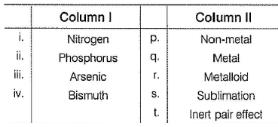Solution:

(i) → (p), (ii) →(q), (iii) → (r,s), (iv)→(q,t)

QUESTION: 17

Match the Column I with Column II and mark the correct option from the codes given below :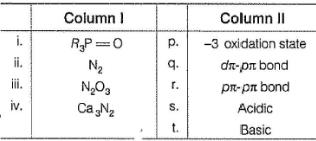Solution:

(i) → (q),(ii) → (r), (iii) → (s), (iv) → (p)

QUESTION: 18

A brown ring is formed in the ring test for NO3 ion. It is due to the formation of

Solution:

When freshly prepared solution of FeSOis added in a solution containing NO3– ion, it leads to formation of a brown coloured complex. This is known as brown ring test of nitrate.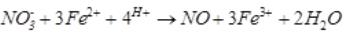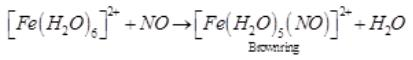*Answer can only contain numeric values
QUESTION: 19

Number of chemical species having negative oxidation state for nitrogen among

NF3, NCI3, NH2OH, NH3,CH3NH2, NH-2, L13N, N20, HCN, HNC, NO-2

Solution:

Explanation : Oxidation state of Nf3 = x+3(-1)= 0

= x-3 = 0

Nf3 = x = 3

NO2 is neutral, so its overall oxidation state is 0. Next, we see that O is more electronegative than N, hence we assign O with the lower oxidation state, of which O usually takes the -2 oxidation state. Let x be the oxidation state of N, hence:

x+2*(-2)=0

x=+4

The oxidation state of N in NO2 is +4.

N2O is nitrous oxide because the oxidation number of the nitrogen is +1.

Oxidation number is the apparent charge on an atom of an element present in a molecule.In NCl3, Electronegativity of Nitrogen is 3 while that of Chlorine is 3.2. Thus Chlorine being more electronegative has oxidation state=-1.

Sum of oxidation numbers of all elements present in a neutral compound is zero.

So, O.N. of Nitrogen + 3(O.N. of Cl)=0

O.N. of N−3 = 0

O.N. of N = +3

*Answer can only contain numeric values
QUESTION: 20

According to molecular orbital theory, number of electrons present in the antibonding orbitals of nitrogen.

Solution: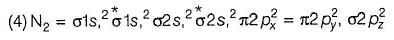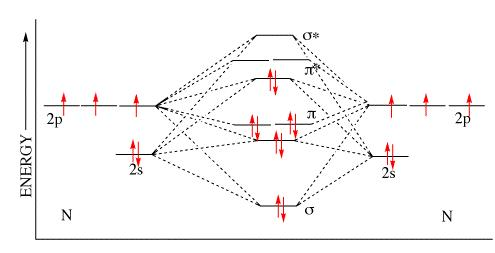QUESTION: 21

Statement Type
This section is based on Statement I and Statement II. Select the correct anser from the codes given below
Statement I N2 is less reactive than P4.
Statement II Nitrogen has more electron gain enthalpy than phosphorus.

Solution:

P — P single bond in Pmolecule is much weaker
(213 kJ mol-1) than N ≡ N triple bond (941.4 kJ mol-1) in N2